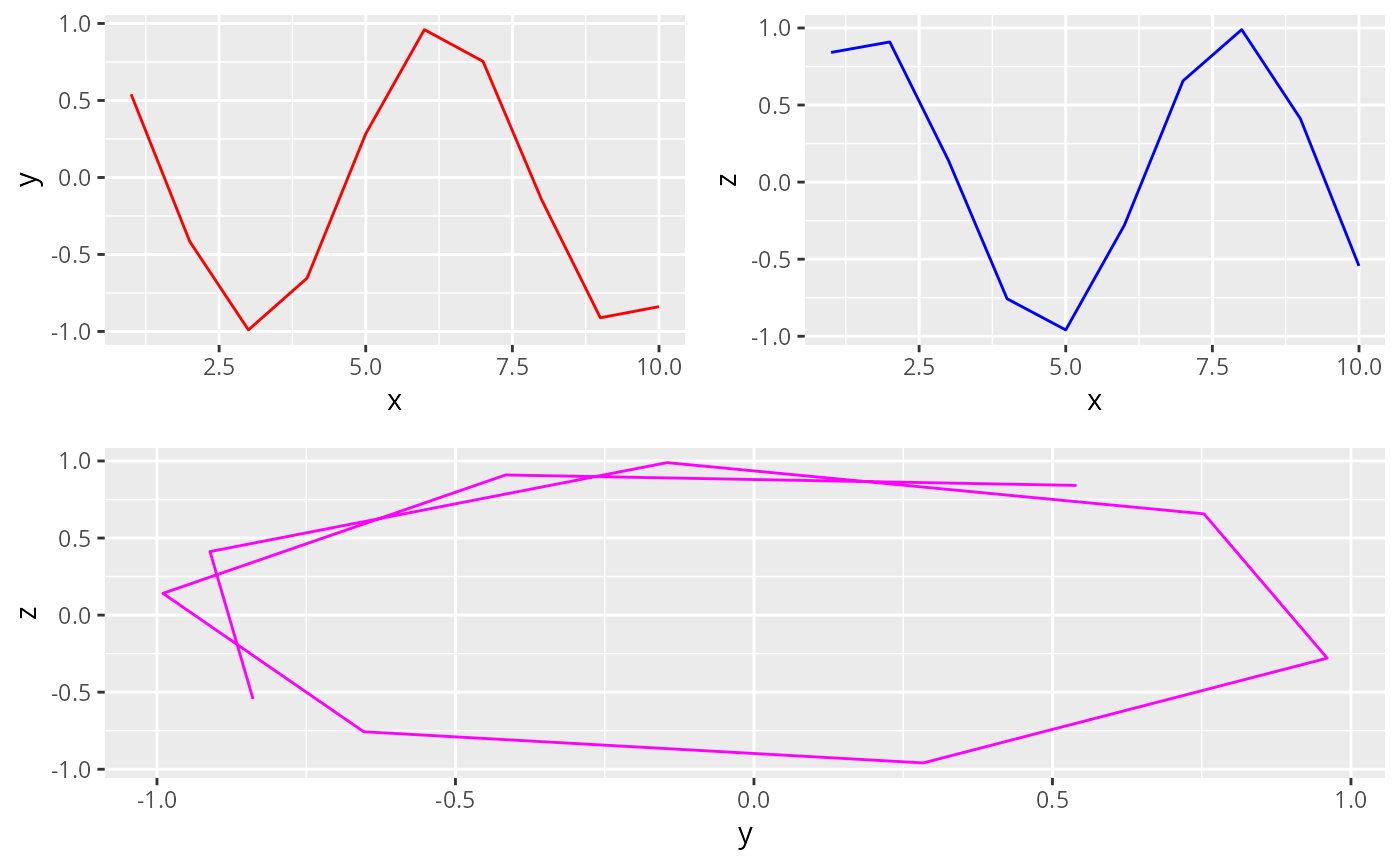Renders multiple ggplots on a single page.

## Usage

multiplot(..., plotlist = NULL, cols = 1, layout = NULL)

## Arguments

...

Comma-separated ggplot objects.

plotlist

A list of ggplot objects - an alternative to the comma-separated argument above.

cols

Number of columns of plots on the page.

layout

A matrix specifying the layout. If present, 'cols' is ignored. If the layout is something like matrix(c(1,2,3,3), nrow=2, byrow=TRUE), then plot 1 will go in the upper left, 2 will go in the upper right, and 3 will go all the way across the bottom.

## Author

David L. Borchers dlb@st-andrews.ac.uk

## Examples

if (require("ggplot2", quietly = TRUE)) {
df <- data.frame(x = 1:10, y = 1:10, z = 11:20)
pl1 <- ggplot(data = df) +
geom_line(mapping = aes(x, y), color = "red")
pl2 <- ggplot(data = df) +
geom_line(mapping = aes(x, z), color = "blue")
multiplot(pl1, pl2, cols = 2)
}